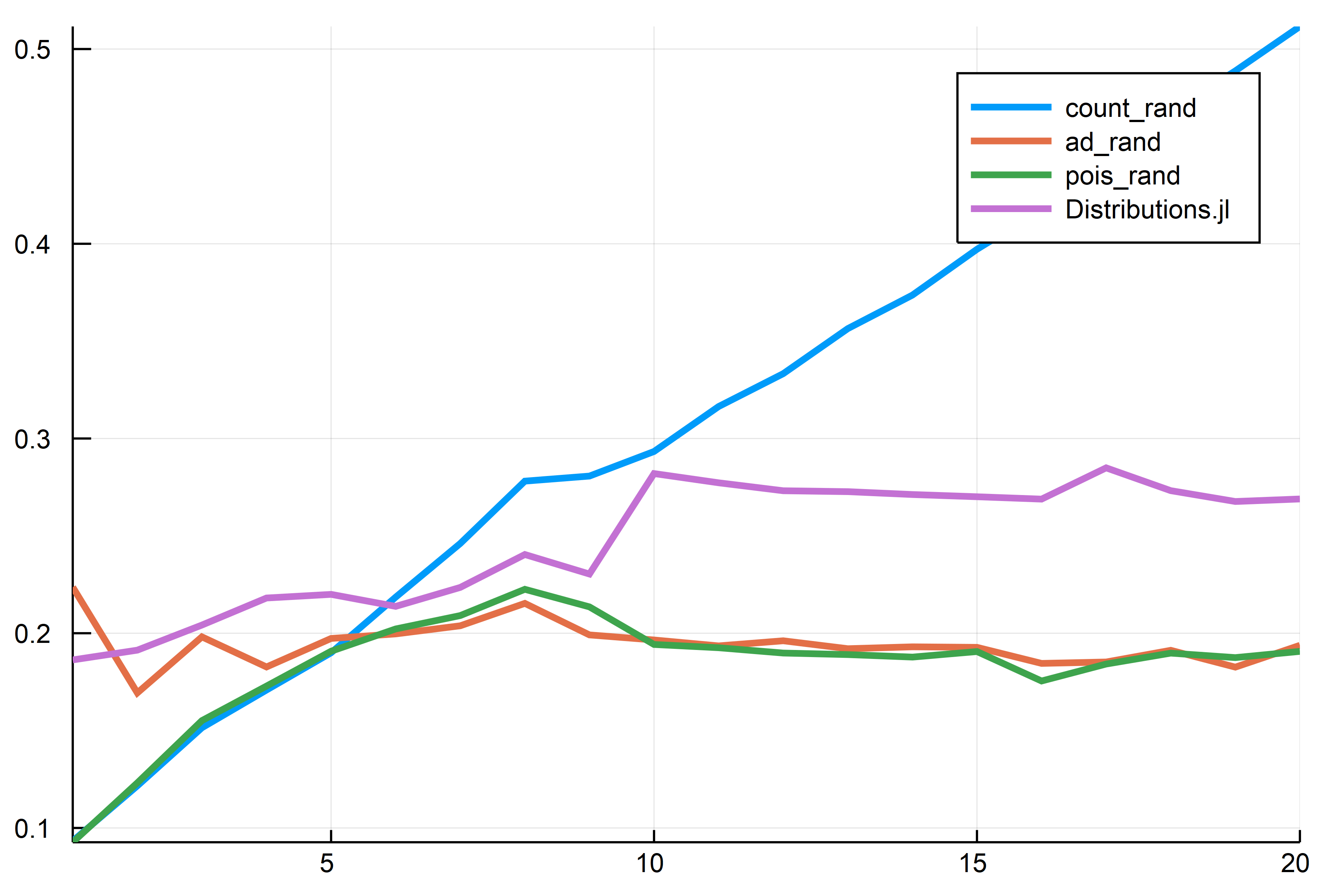# PoissonRandom.jl: Fast Poisson Random Numbers

PoissonRandom.jl is a component of the SciML ecosystem which allows for fast generation of Poisson random numbers.

## Installation

To install ParameterizedFunctions.jl, use the Julia package manager:

using Pkg
Pkg.add("PoissonRandom")

## Usage

# Simple Poisson random
pois_rand(λ)

# Using another RNG
using RandomNumbers
rng = Xorshifts.Xoroshiro128Plus()
pois_rand(rng,λ)

## Implementation

It mixes two methods. A simple count method and a method from a normal approximation. See this blog post for details.

## Benchmark

using RandomNumbers, Distributions, BenchmarkTools, StaticArrays,
RecursiveArrayTools, Plots, PoissonRandom
rng = Xorshifts.Xoroshiro128Plus()

function n_count(rng,λ,n)
tmp = 0
for i in 1:n
tmp += PoissonRandom.count_rand(rng,λ)
end
end

function n_pois(rng,λ,n)
tmp = 0
for i in 1:n
tmp += pois_rand(rng,λ)
end
end

tmp = 0
for i in 1:n
end
end

function n_dist(λ,n)
tmp = 0
for i in 1:n
tmp += rand(Poisson(λ))
end
end

function time_λ(rng,λ,n)
t1 = @elapsed n_count(rng,λ,n)
t3 = @elapsed n_pois(rng,λ,n)
t4 = @elapsed n_dist(λ,n)
@SArray [t1,t2,t3,t4]
end

# Compile
time_λ(rng,5,5000000)
# Run with a bunch of λ
times = VectorOfArray([time_λ(rng,n,5000000) for n in 1:20])'
plot(times,labels = labels, lw = 3)So this package ends up about 30% or so faster than Distributions.jl (the method at the far edge is λ-independent so that goes on forever).